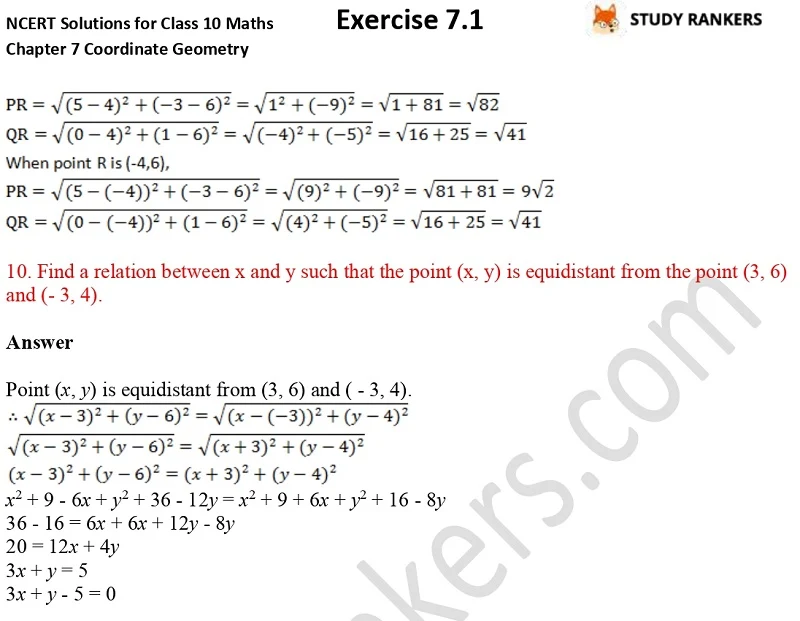## NCERT Solutions for Class 10 Maths Chapter 7 Coordinate Geometry Exercise 7.1

Class 10 students wants accurate Class 10 Maths NCERT Solutions thus we have provided Chapter 7 Coordinate Geometry Exercise 7.1 NCERT Solutions so you can get step by step solutions to every problems. These will be very useful in completing your homework and scoring better marks in the examinations. CBSE NCERT Solutions prepared by Studyrankers experts are updated as per 2019-20.

There are 10 questions in the exercise in which variety of questions such as find the distance between the given pairs of points, find the values of y for which the distance between the points P (2, - 3) and Q (10, y) is 10 units etc.